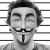## 想兼职的朋友来看看~

pinksalley 发布于 2011/11/10 10:27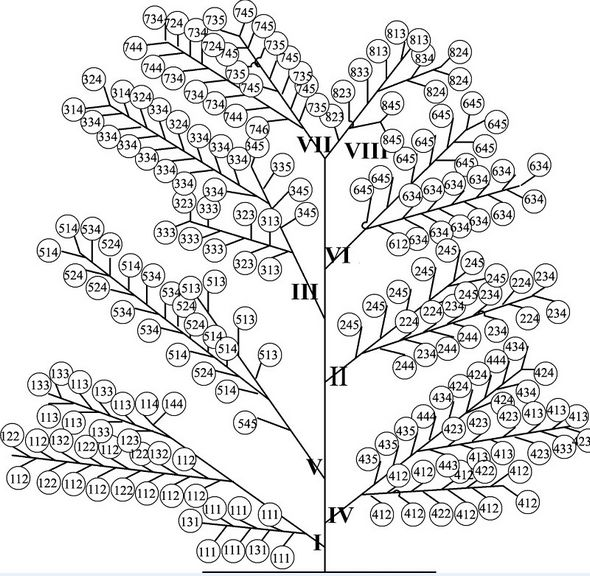00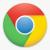```public class Leaf implements Comparable<Leaf> {
private String name;

public Leaf(String number) {
this.name = number;
}

public int compareTo(Leaf leaf) {
int firstComp = this.getName().substring(0, 1).compareTo(
leaf.getName().substring(0, 1));
if (firstComp == 0) {
return this.getName().substring(2, 3).compareTo(
leaf.getName().substring(2, 3));
} else {
return firstComp;
}
}

public String getName() {
return name;
}
}

import java.util.ArrayList;
import java.util.Collections;
import java.util.Iterator;
import java.util.List;

public class Tree {

public static void main(String[] args) {
Leaf lf1=new Leaf("123");
Leaf lf2=new Leaf("122");
Leaf lf11=new Leaf("623");
Leaf lf13=new Leaf("621");
Leaf lf14=new Leaf("653");
Leaf lf12=new Leaf("659");
Leaf lf3=new Leaf("223");
Leaf lf4=new Leaf("223");
Leaf lf5=new Leaf("323");
Leaf lf9=new Leaf("523");
Leaf lf10=new Leaf("524");
Leaf lf6=new Leaf("323");
Leaf lf7=new Leaf("125");
Leaf lf8=new Leaf("128");
List<Leaf> tree=new ArrayList<Leaf>() ;
Collections.sort(tree);
Iterator<Leaf> iter=tree.iterator();
while(iter.hasNext()){
String number=iter.next().getName();
String unit=number.substring(0,1);
System.out.println("第"+unit+"單元編號："+number);
}
}

}```

0
w

0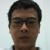0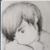0